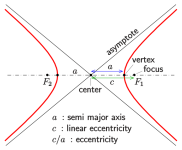# How calculate semi-latus rectum of parabola or hyperbola?

#### Kolodez

##### New member
The semi-latus rectum is necessary for the formula
radius = semi-latus rectum / (1 + eccentricity * cos (true anomaly)).
However, if we refer to Wikipedia, we need to know the semi-major axis to calculate the semi-latus rectum. But the semi-major axis is only defined for circles and ellipses, if I am not getting it wrong.
So assume I am in a parabolic or hyperbolic orbit and I know my current location, velocity, true anomaly, specific energy, eccentricity and the periapsis radius. How can I calculate the semi-latus rectum from these values in order to be able to calculate future locations?
Thank you!

#### n72.75

##### Move slow and break things
Orbiter Contributor
Tutorial Publisher
Donator
Semi-major axis is defined for hyperbolae and parabolae.#### Sbb1413

##### Well-known member
But the semi-major axis is only defined for circles and ellipses, if I am not getting it wrong.
AFAIK semi-major axis is defined for any conic sections, not just circles and ellipses, as @n72.75 has already pointed out.

#### Kolodez

##### New member
Thanks for your replies! So if I have a hyperbola, I can also use the formula
semi-major axis = - G * major body mass / (2 * orbit specific energy)
and the semi-major axis is then negative, whereas it is positive in the case of an ellipse, right? And that yields the same formula for the semi-latus rectum, i.e.
semi-latus rectum = semi-major axis * (1 - eccentricity^2)
no matter if we have an ellipse or a hyperbola.
But in the case of a parabola, the above formulas lead to
semi-major-axis = infinity,
semi-latus rectum = infinity * 0
which makes impossible to calculate the semi-latus rectum. Did I make an error anywhere?

#### Kolodez

##### New member
Oh, now I saw the formula
Semi-latus rectum = 2 * periapsis radius
But to compute the right hand side, I only know the formula
periapsis radius = semi-major axis * (1 - eccentricity)
which also seems to be undefined in the case of a parabola. So I still have no idea what to do in the case of a parabola.

#### jarmonik

##### Well-known member
Orbiter Contributor
Beta Tester
Semi-latus rectum = 2 * periapsis radius
This is wrong.

periapsis radius = Semi-latus rectum / ( 1 + eccentricity )

Parabola in orbital mechanics in the Orbiter is something that you can forget. Doesn't really exists in practice.

#### Kolodez

##### New member
@jarmonik, this is correct if eccentricity = 1. This is what I meant, sorry.

#### Kolodez

##### New member
I looked into the Orbiter manual once again. We have
semi-major axis = - G * major body mass / (2 * orbit specific energy),
eccentricity = sqrt (1 + 2 * orbit specific energy * h^2 / (G^2 * major body mass^2))

Hence
semi-latus rectum = semi-major axis * (1 - eccentricity^2)
= - G * major body mass / (2 * orbit specific energy) * ( - 2 * orbit specific energy * h^2 / (G^2 * major body mass^2))
= h^2 / (G * major body mass)

where h = radius x velocity. Since everything is continuous, we can use this for any eccentricity. Is this correct?

#### jarmonik

##### Well-known member
Orbiter Contributor